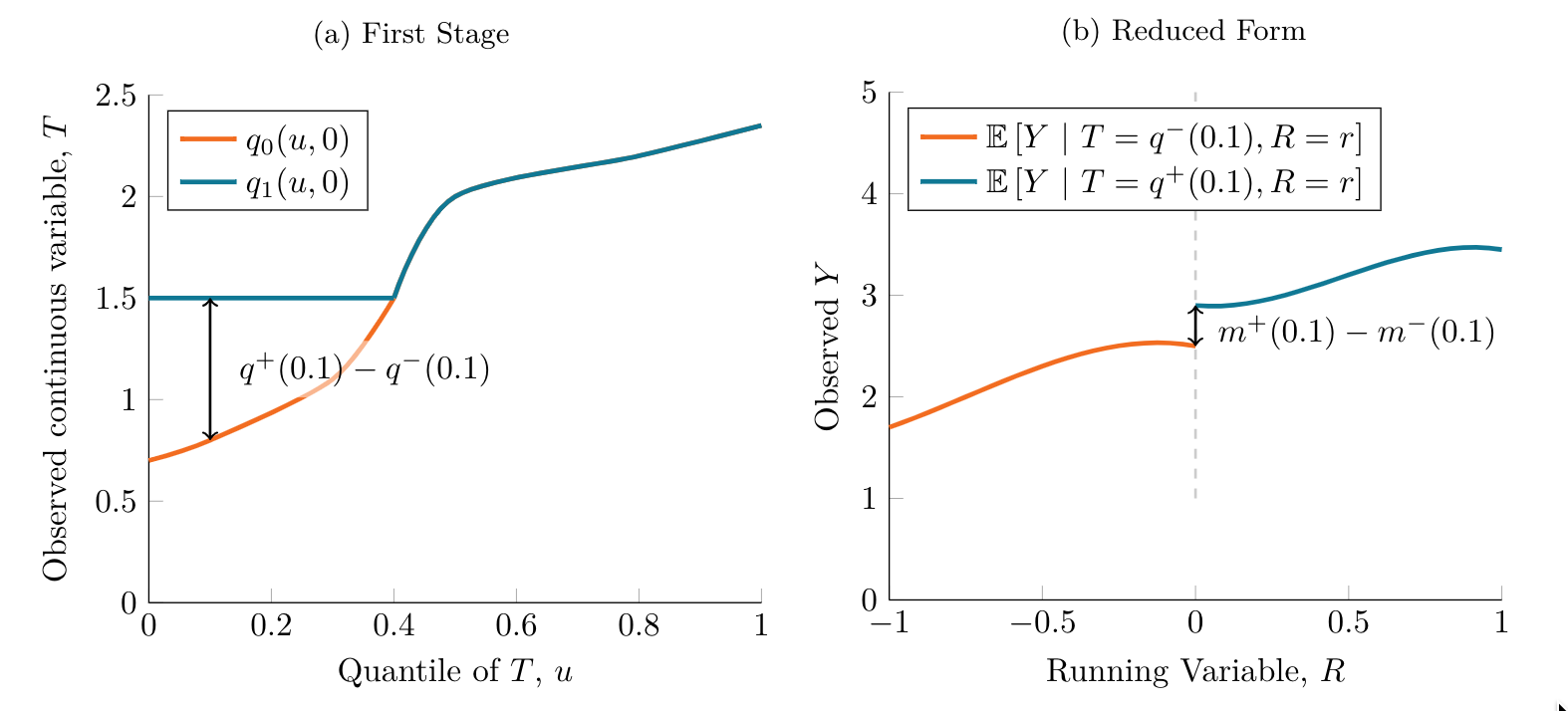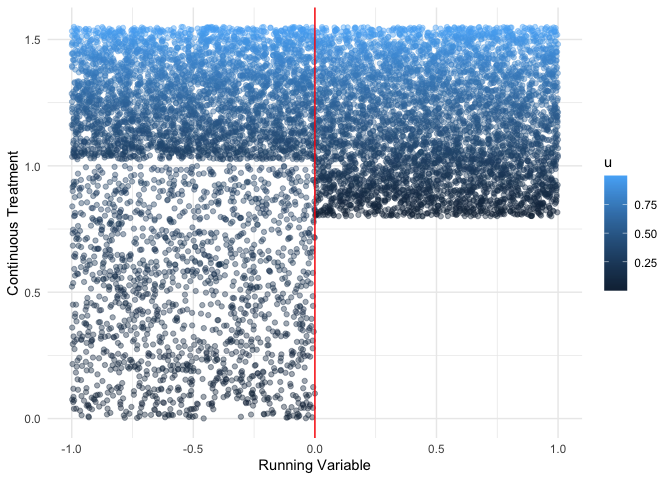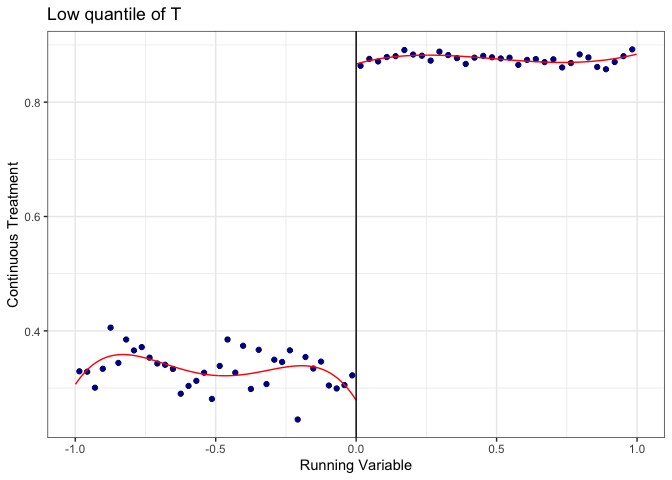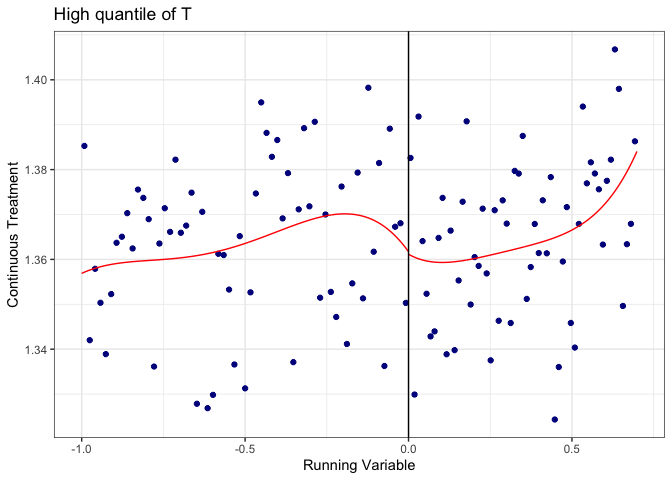# ContinuousRD

ContinuousRD is a package that implements regression discontinuity designs when the treatment variable is continuous following Dong, Lee, and Gou (2022)

## Installation

You can install the development version of ContinuousRD from GitHub with:

r# install.packages("devtools")devtools::install_github("kylebutts/ContinuousRD")

## Setting

I will provide a quick (and simplified) overview of the methods from Dong, Lee, and Gou (2022). For the full details, please see the original text. I’m purposefully leaving out details since hopefully you are here after reading the paper :-)

Let $Y$ denote the outcome variable and $T$ a continuous variable of interest. There exists a running variable $R$ and a cutoff $c$ (which we’ll normalize to zero) that affects the treatment $T$. The paper assumes that different quantiles of $T$ are differentially affected by the discontinuity.

For example, in the paper they discuss a minimum capital requirement for banks in cities with population over 3,000 in 1900. The running variable would be population and the treatment variable is banking capital. Clearly, only banks with counterfactual banking capital below the threshold are affected by the population cutoff. Therefore the jump in $T$ will be zero for most banks, but positive for banks at the lower end of the counterfactual distribution of $T$.

For this reason, the estimator proposed in the paper wants to estimate the LATE at different quantiles of the distribution of $T$, which they denote as the Quantile-LATE or QLATE for short. The method proceeds as follows.

For a given quantile of $T$, denoted $u \in (0,1)$, estimate the “first-stage” RD of $T$. Define $q^+(u)$ be the $u^{th}$ percentile of $T$ under treatment at $R = 0$ and $q^-(u)$ be the $u^{th}$ percentile of $T$ under no treatment at $R = 0$. The RD estimate of $T$ would therefore estimate This will estimate $\mathbb{E}[ q^+(u) - q^-(u) \vert U = u, R = 0]$. Intuitively, this gives the jump in the continuous treatment for units at the $u^{th}$ percentile of the distribution.

Then, the reduced form involves running an RD of $Y$ using observations to the right of the cutoff with values of $T$ near $T_1(u)$ and to the left of the cutoff with values of $T$ near $T_0(u)$. The left and right limits in the RD would respectively identify

$m^-(u) = \mathbb{E}[ Y | T = q^-(u), R = 0] \text{ and } m^+(u) = \mathbb{E}[ Y | T = q^+(u), R = 0]$

The difference between these two terms would estimate the jump in $Y$ for units near the $u^{th}$ percentile of $T$. The QLATE estimate will then be the ratio of these two quantities. Intuitively it’s the jump in $Y$ rescaled by the jump in $T$. Note that only quantiles that have a significant jump $q^+(u) - q^-(u) \neq 0$ are used to form estimates (since otherwise the denominator is 0).A visual example using a made-up DGP similar to the paper’s main example is shown here for a given quantile $u = 0.1$. The left-figure shows the estimated jump in $T$. The right-figure shows the estimated jump in $Y$ at value of $q^-(u)$ and $q^+(u)$. The ratio of these two estimates would be the estimated treatment effect and can be interpreted as the jump in $Y$ per unit increase in $T$.

## Example

I will use a set of simulated data as an illustration of the method

rlibrary(ContinuousRD)#> Loading required package: data.table# Load datadata(sim_data)head(sim_data)#> Key: <id>#>       id         u           R     Z         T         Y#>    <int>     <num>       <num> <num>     <num>     <num>#> 1:     1 0.2002145 -0.71892650     0 0.6673815 0.6652777#> 2:     2 0.6852186  0.19781138     1 1.3139139 1.3141626#> 3:     3 0.9168758  0.03111523     1 1.4876568 1.4881608#> 4:     4 0.2843995  0.64044390     1 1.0132996 1.0165819#> 5:     5 0.1046501 -0.75736198     0 0.3488338 0.3474286#> 6:     6 0.7010575  0.06593254     1 1.3257931 1.3291840

The data consists of 10000 observations each of which is a unique unit. The variable Y is the outcome variable, T is the continuous treatment of interest, R is the running variable with a cutoff of $0$. Positive values of the running variable are affected by the border, in this case a binding lower bound on T. The variable u is the (typically unobserved) percentile of each unit for the variable T.

The below data shows the observed continuous treatment along the running variable. The color of the dot represents the quantile of T at that value of R. You can see a clear jump at the border where T is affected by the binding constraint.

rlibrary(ggplot2)ggplot(sim_data) +    geom_point(        aes(x = R, y = T, color = u),        alpha = 0.4    ) +     geom_vline(xintercept = 0, color = "red") +    labs(        x = "Running Variable",        y = "Continuous Treatment"    ) +    theme_minimal()However, this is only binding for units at the lower end of the distribution. To see this more clearly, let’s look at multiple RDs at different quantiles of T.

rlibrary(rdrobust)low_q = sim_data[sim_data$u > 0 & sim_data$u < 0.2,]high_q = sim_data[sim_data$u > 0.5 & sim_data$R < 0.7,]rdrobust::rdplot(    y = low_q$T, x = low_q$R,     title = "Low quantile of T", y.label = "Continuous Treatment", x.label = "Running Variable")rrdrobust::rdplot(    y = high_q$T, x = high_q$R,     title = "High quantile of T", y.label = "Continuous Treatment", x.label = "Running Variable")The data is simulated to have units with quantile below $0.3$ be affected by the cutoff (the magnitude of the change in T depends on quantile u) and those units experience a treatment effect of size $1$. Let’s see if we get that using the QLATE_bc_se function:

rres <- ContinuousRD::QLATE_bc_se(sim_data, qlist = seq(0.1, 0.9, by = 0.1), yname = "Y", tname = "T", rname = "R", c = 0)#> qlist: 0.1, 0.2, 0.3, 0.4, 0.5, 0.6, 0.7, 0.8, and 0.9#> tau_u: 1, 1.001, NA, NA, NA, NA, NA, NA, and NA#> SEtau: 0.005, 0.018, NA, NA, NA, NA, NA, NA, and NA#> tau_u_bc: 0.992, 0.985, NA, NA, NA, NA, NA, NA, and NA#> SEtau_bc: 2.384, 7.942, NA, NA, NA, NA, NA, NA, and NA#> pi: 1.001#> SEpi: 0.004#> pi_bc: 0.991#> SEpi_bc: 0.359

In the printed results a value of NA appears when no significant jump in the treatment variable is found at the cutoff. In this case, since the jump around the 30th percentile is near zero, it doesn’t show up in the estimate. However, we see there the effect is around $1$ as expected for the 10th and 20th percentile. The overall average effect pi is also approximately $1$ as well.